Скачать презентацию CAP 6135 Malware and Software Vulnerability Analysis Basic

7644e5c7b16876e7c7836c5f6a6208e5.ppt

• Количество слайдов: 48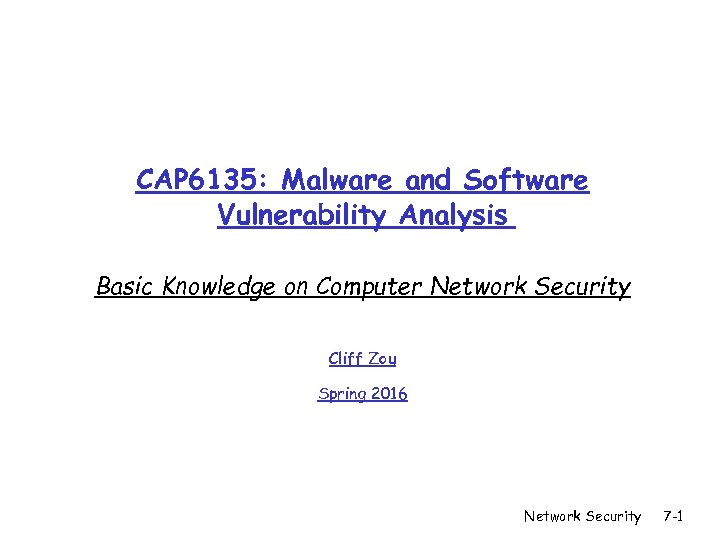CAP 6135: Malware and Software Vulnerability Analysis Basic Knowledge on Computer Network Security Cliff Zou Spring 2016 Network Security 7 -1Acknowledgement r This lecture note is modified from the slides provided by textbook: v Computer Networking: A Top Down Approach Featuring the Internet, J. Kurose & K. Ross, Addison Wesley, 5 rd ed. , 2009 Network Security 7 -2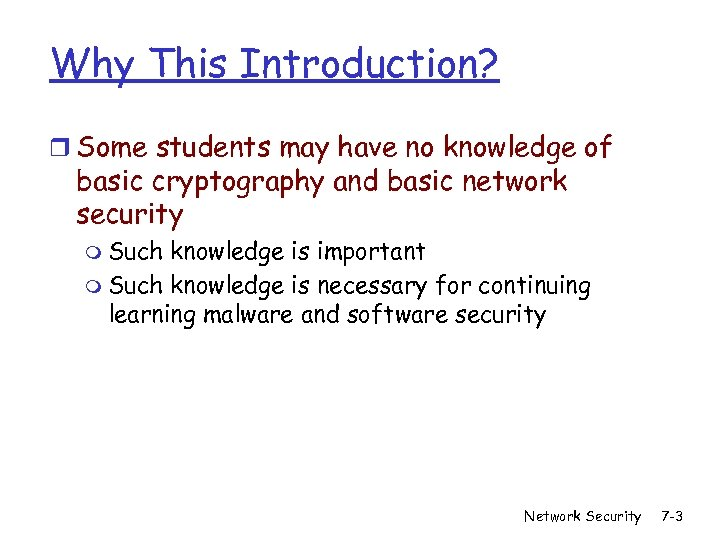Why This Introduction? r Some students may have no knowledge of basic cryptography and basic network security m Such knowledge is important m Such knowledge is necessary for continuing learning malware and software security Network Security 7 -3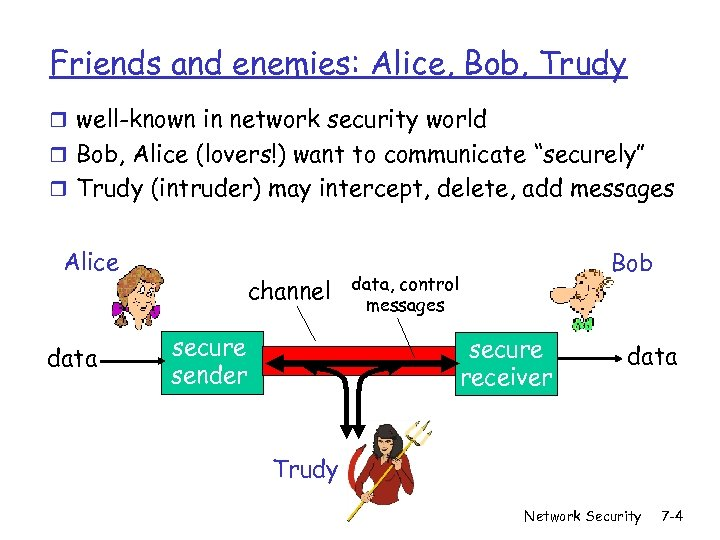Friends and enemies: Alice, Bob, Trudy r well-known in network security world r Bob, Alice (lovers!) want to communicate “securely” r Trudy (intruder) may intercept, delete, add messages Alice data channel secure sender Bob data, control messages secure receiver data Trudy Network Security 7 -4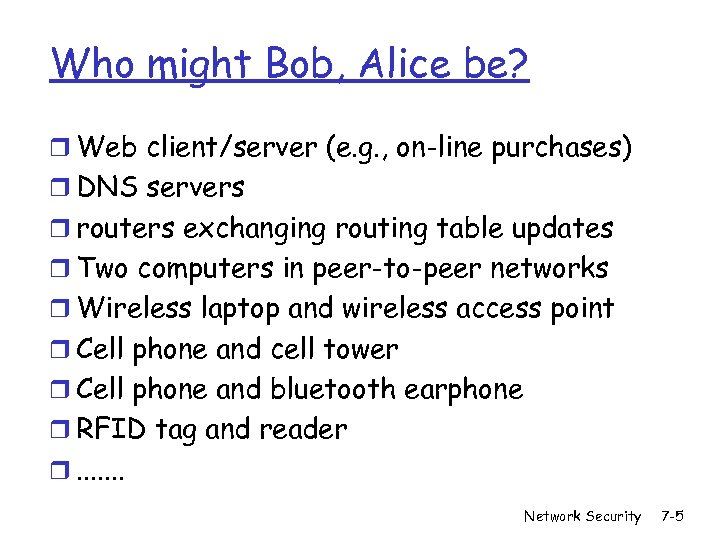Who might Bob, Alice be? r Web client/server (e. g. , on-line purchases) r DNS servers r routers exchanging routing table updates r Two computers in peer-to-peer networks r Wireless laptop and wireless access point r Cell phone and cell tower r Cell phone and bluetooth earphone r RFID tag and reader r. . . . Network Security 7 -5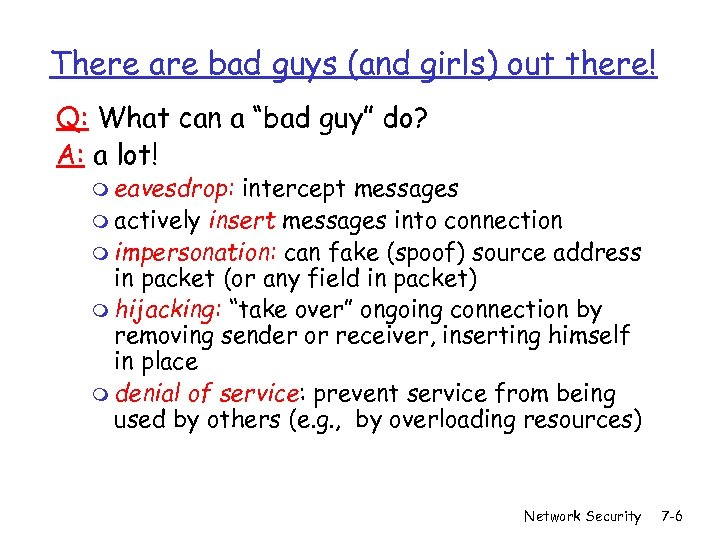There are bad guys (and girls) out there! Q: What can a “bad guy” do? A: a lot! m eavesdrop: intercept messages m actively insert messages into connection m impersonation: can fake (spoof) source address in packet (or any field in packet) m hijacking: “take over” ongoing connection by removing sender or receiver, inserting himself in place m denial of service: prevent service from being used by others (e. g. , by overloading resources) Network Security 7 -6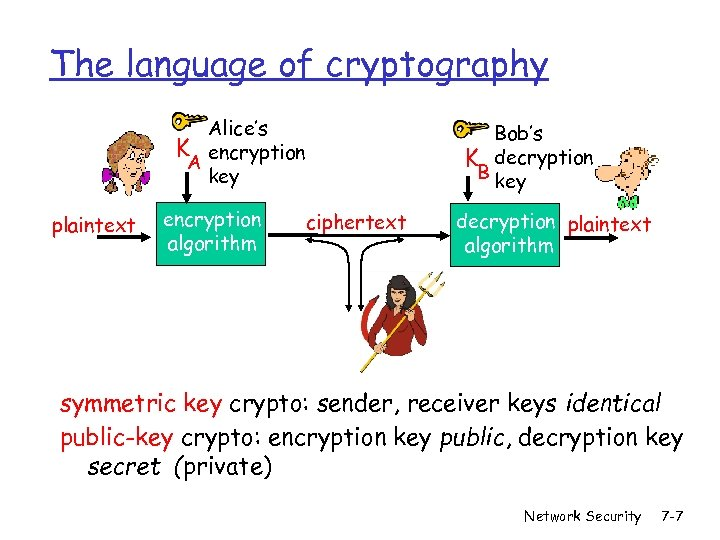The language of cryptography Alice’s K encryption A key plaintext encryption algorithm Bob’s K decryption B key ciphertext decryption plaintext algorithm symmetric key crypto: sender, receiver keys identical public-key crypto: encryption key public, decryption key secret (private) Network Security 7 -7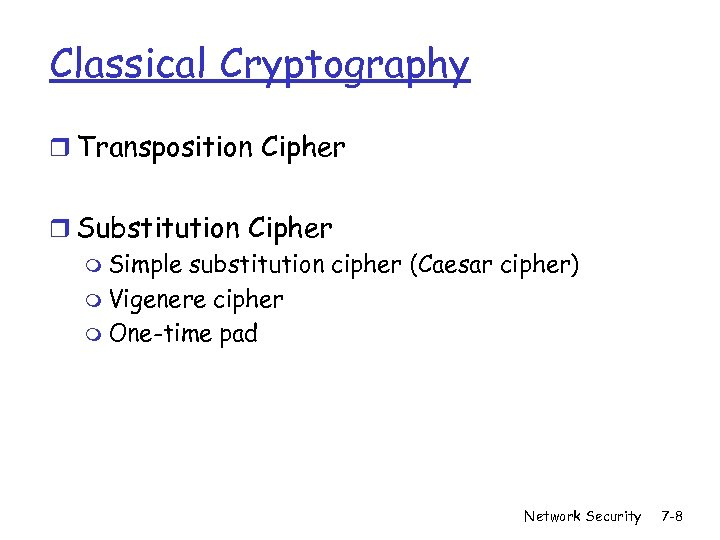Classical Cryptography r Transposition Cipher r Substitution Cipher m Simple substitution cipher (Caesar cipher) m Vigenere cipher m One-time pad Network Security 7 -8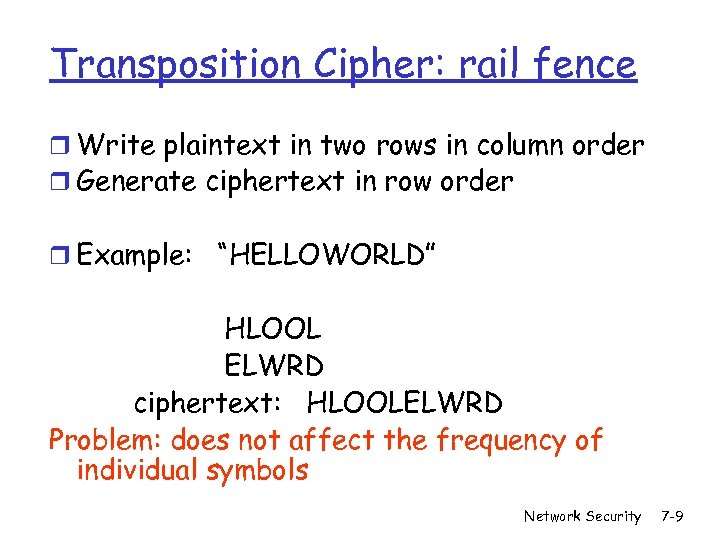Transposition Cipher: rail fence r Write plaintext in two rows in column order r Generate ciphertext in row order r Example: “HELLOWORLD” HLOOL ELWRD ciphertext: HLOOLELWRD Problem: does not affect the frequency of individual symbols Network Security 7 -9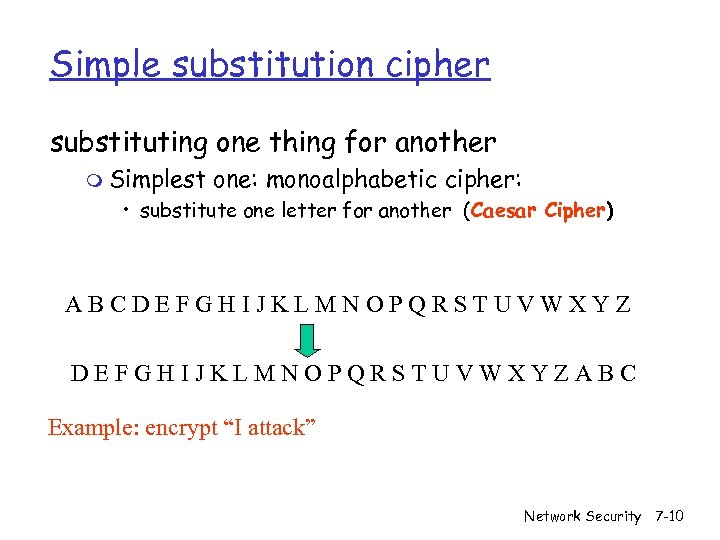Simple substitution cipher substituting one thing for another m Simplest one: monoalphabetic cipher: • substitute one letter for another (Caesar Cipher) ABCDEFGHIJKLMNOPQRSTUVWXYZABC Example: encrypt “I attack” Network Security 7 -10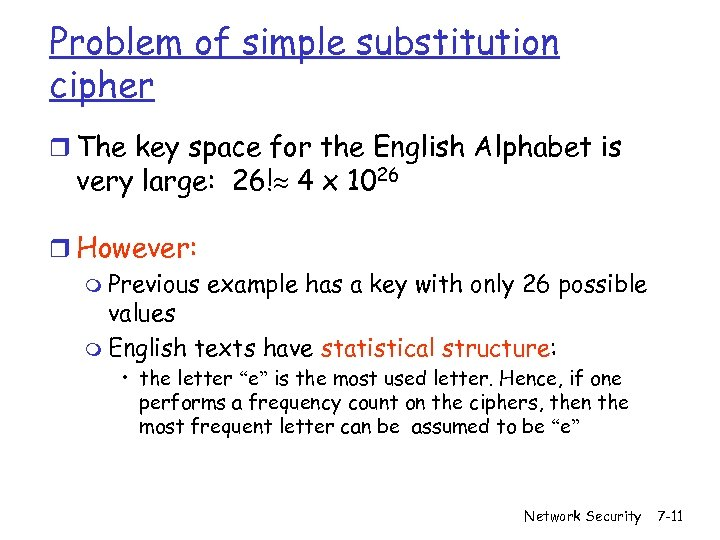Problem of simple substitution cipher r The key space for the English Alphabet is very large: 26! 4 x 1026 r However: m Previous example has a key with only 26 possible values m English texts have statistical structure: • the letter “e” is the most used letter. Hence, if one performs a frequency count on the ciphers, then the most frequent letter can be assumed to be “e” Network Security 7 -11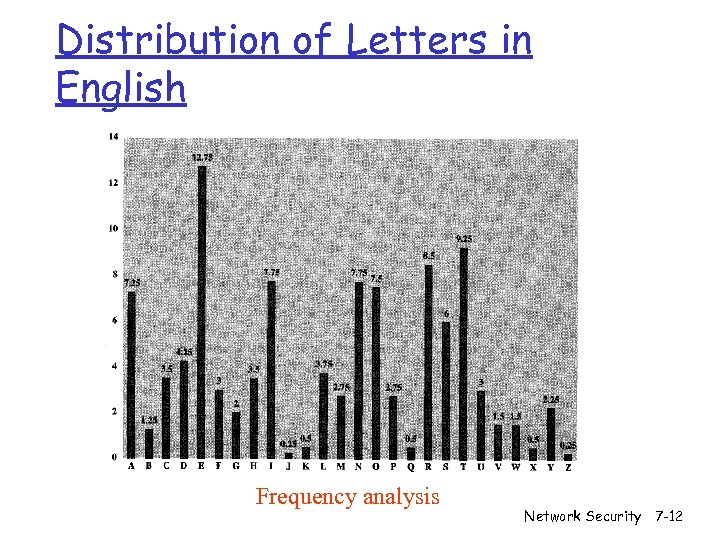Distribution of Letters in English Frequency analysis Network Security 7 -12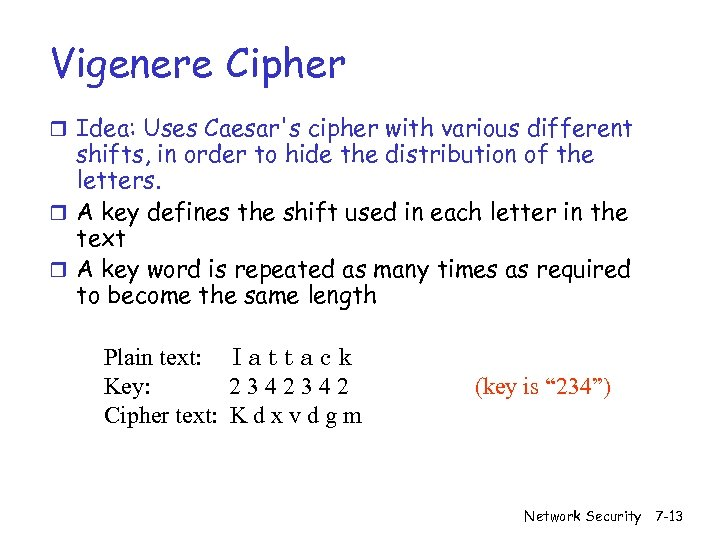Vigenere Cipher r Idea: Uses Caesar's cipher with various different shifts, in order to hide the distribution of the letters. r A key defines the shift used in each letter in the text r A key word is repeated as many times as required to become the same length Plain text: I a t t a c k Key: 2342342 Cipher text: K d x v d g m (key is “ 234”) Network Security 7 -13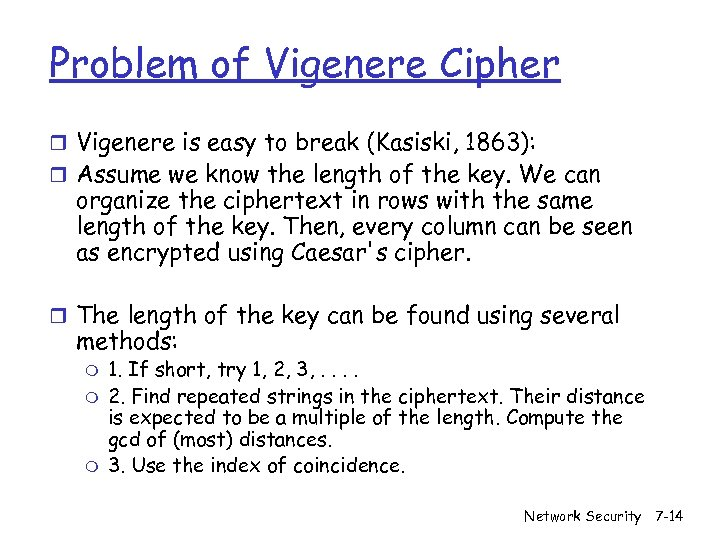Problem of Vigenere Cipher r Vigenere is easy to break (Kasiski, 1863): r Assume we know the length of the key. We can organize the ciphertext in rows with the same length of the key. Then, every column can be seen as encrypted using Caesar's cipher. r The length of the key can be found using several methods: m m m 1. If short, try 1, 2, 3, . . 2. Find repeated strings in the ciphertext. Their distance is expected to be a multiple of the length. Compute the gcd of (most) distances. 3. Use the index of coincidence. Network Security 7 -14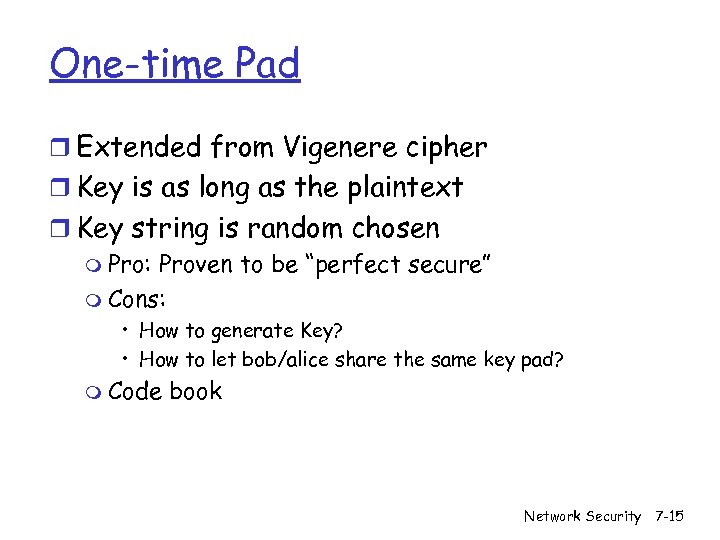One-time Pad r Extended from Vigenere cipher r Key is as long as the plaintext r Key string is random chosen m Pro: Proven to be “perfect secure” m Cons: • How to generate Key? • How to let bob/alice share the same key pad? m Code book Network Security 7 -15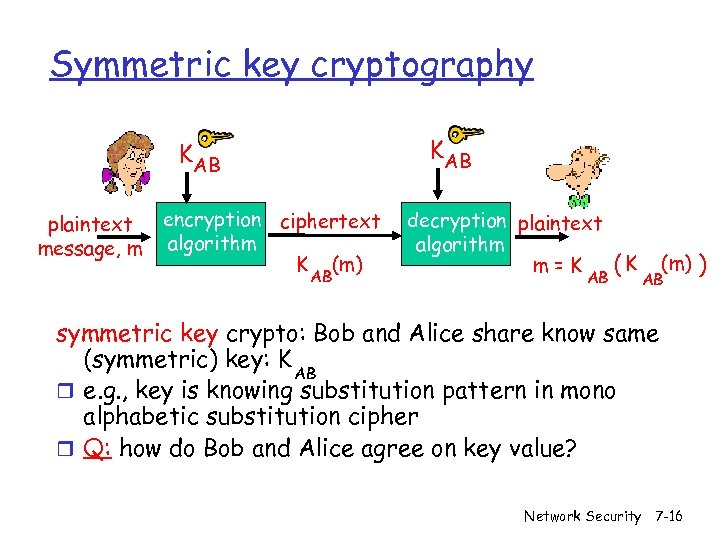Symmetric key cryptography KAB plaintext message, m encryption ciphertext algorithm K (m) AB decryption plaintext algorithm m=K AB ( K (m) ) AB symmetric key crypto: Bob and Alice share know same (symmetric) key: K AB r e. g. , key is knowing substitution pattern in mono alphabetic substitution cipher r Q: how do Bob and Alice agree on key value? Network Security 7 -16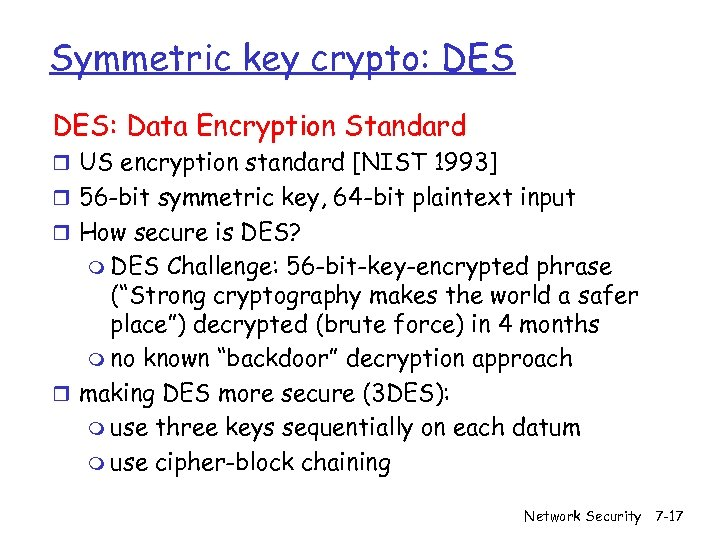Symmetric key crypto: DES: Data Encryption Standard r US encryption standard [NIST 1993] r 56 -bit symmetric key, 64 -bit plaintext input r How secure is DES? m DES Challenge: 56 -bit-key-encrypted phrase (“Strong cryptography makes the world a safer place”) decrypted (brute force) in 4 months m no known “backdoor” decryption approach r making DES more secure (3 DES): m use three keys sequentially on each datum m use cipher-block chaining Network Security 7 -17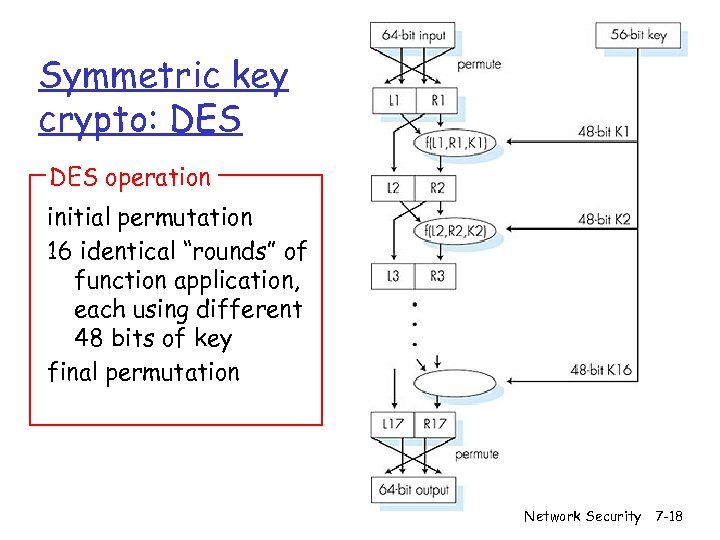Symmetric key crypto: DES operation initial permutation 16 identical “rounds” of function application, each using different 48 bits of key final permutation Network Security 7 -18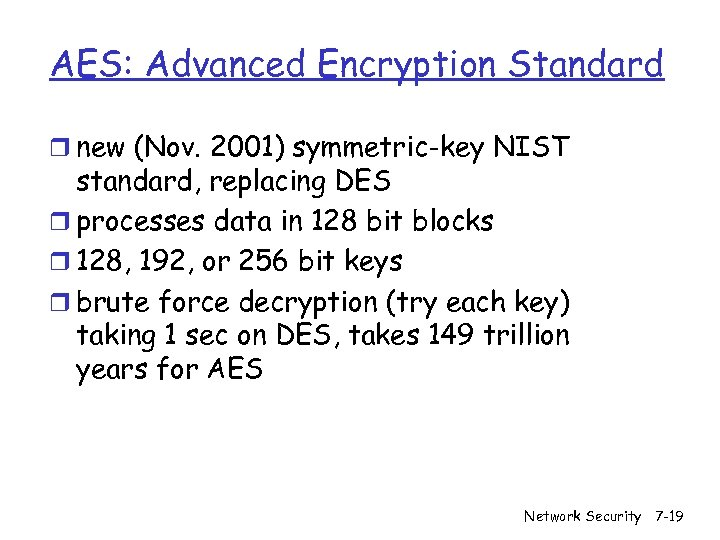AES: Advanced Encryption Standard r new (Nov. 2001) symmetric-key NIST standard, replacing DES r processes data in 128 bit blocks r 128, 192, or 256 bit keys r brute force decryption (try each key) taking 1 sec on DES, takes 149 trillion years for AES Network Security 7 -19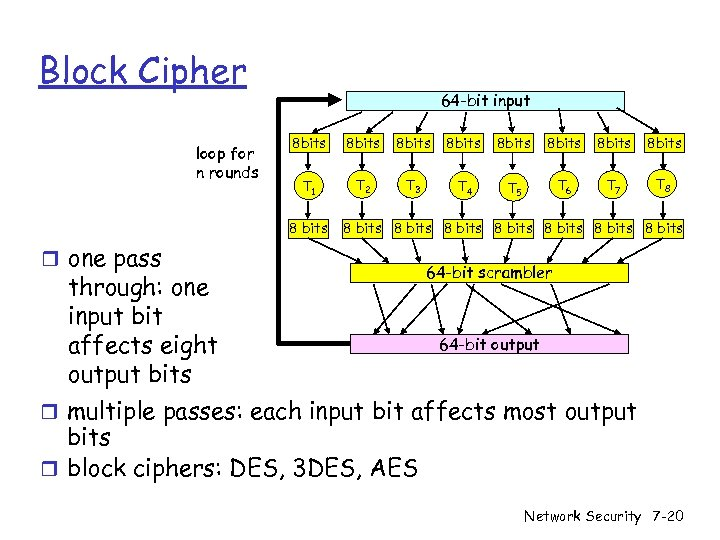Block Cipher loop for n rounds 64 -bit input 8 bits 8 bits T 1 T 2 T 3 T 4 T 5 T 6 T 7 T 8 8 bits 8 bits r one pass through: one input bit affects eight output bits 64 -bit scrambler 64 -bit output r multiple passes: each input bit affects most output bits r block ciphers: DES, 3 DES, AES Network Security 7 -20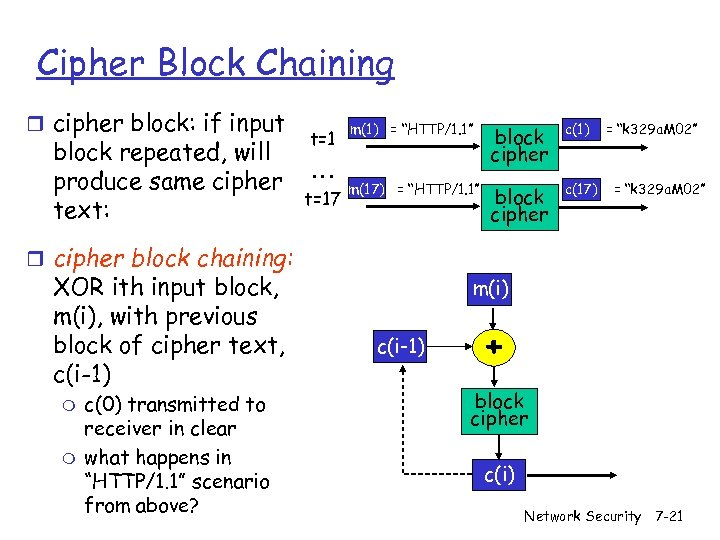Cipher Block Chaining r cipher block: if input block repeated, will produce same cipher text: t=1 … t=17 m(1) = “HTTP/1. 1” block cipher c(1) m(17) = “HTTP/1. 1” block cipher c(17) = “k 329 a. M 02” r cipher block chaining: XOR ith input block, m(i), with previous block of cipher text, c(i-1) m m c(0) transmitted to receiver in clear what happens in “HTTP/1. 1” scenario from above? m(i) c(i-1) + block cipher c(i) Network Security 7 -21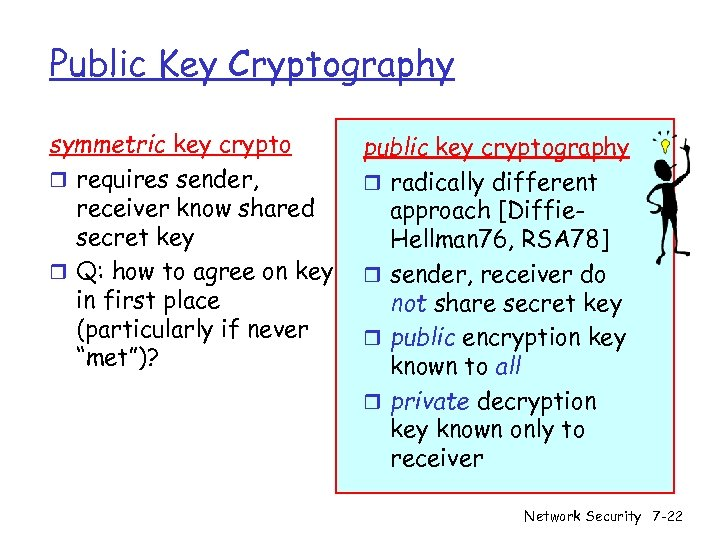Public Key Cryptography symmetric key crypto r requires sender, receiver know shared secret key r Q: how to agree on key in first place (particularly if never “met”)? public key cryptography r radically different approach [Diffie. Hellman 76, RSA 78] r sender, receiver do not share secret key r public encryption key known to all r private decryption key known only to receiver Network Security 7 -22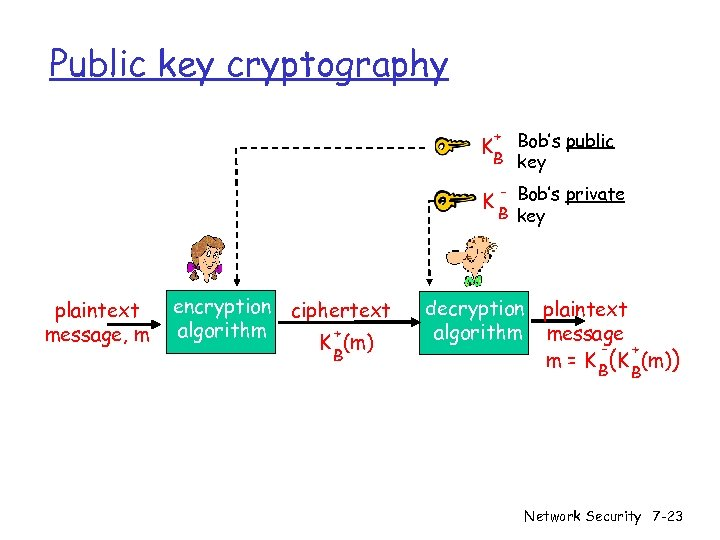Public key cryptography + Bob’s public B key K K plaintext message, m encryption ciphertext algorithm + K (m) B - Bob’s private B key decryption plaintext algorithm message + m = K B(K (m)) B Network Security 7 -23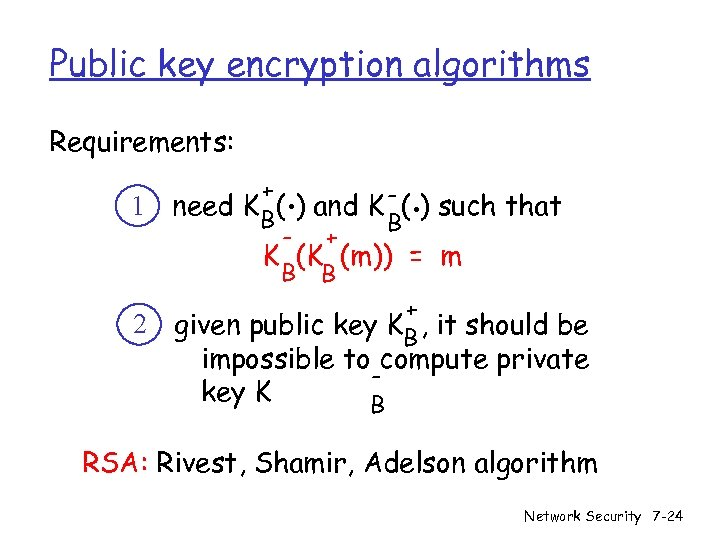Public key encryption algorithms Requirements: 1 2 . . + need K B( ) and K - ( ) such that B - + K (K (m)) = m B B + given public key KB , it should be impossible to compute private key K B RSA: Rivest, Shamir, Adelson algorithm Network Security 7 -24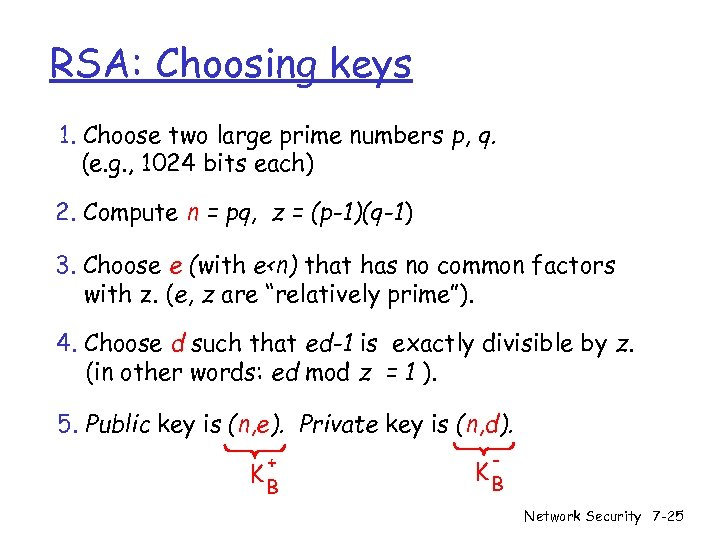RSA: Choosing keys 1. Choose two large prime numbers p, q. (e. g. , 1024 bits each) 2. Compute n = pq, z = (p-1)(q-1) 3. Choose e (with e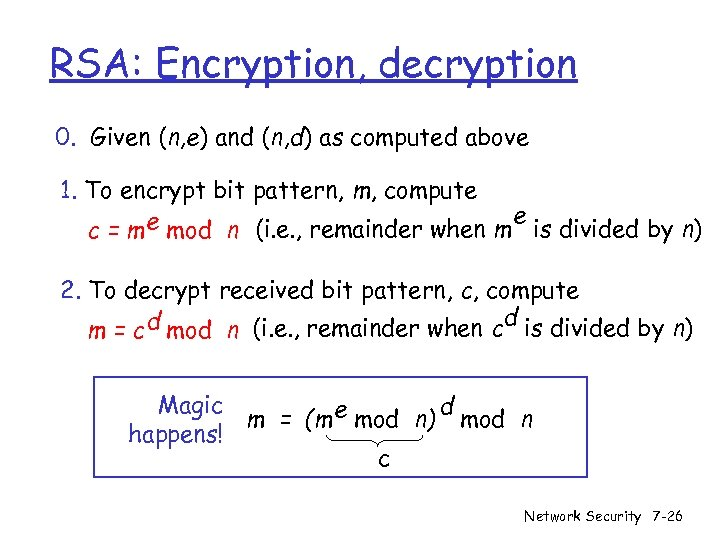RSA: Encryption, decryption 0. Given (n, e) and (n, d) as computed above 1. To encrypt bit pattern, m, compute e mod n (i. e. , remainder when m e is divided by n) c=m 2. To decrypt received bit pattern, c, compute d m = c d mod n (i. e. , remainder when c is divided by n) Magic d m = (m e mod n) mod n happens! c Network Security 7 -26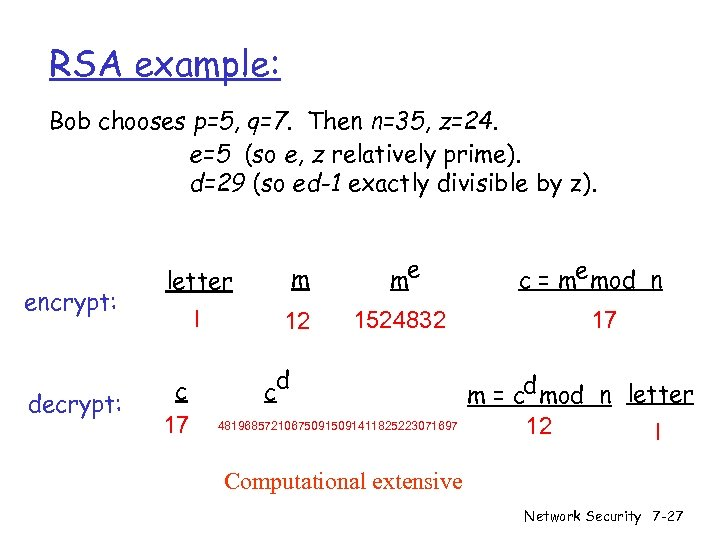RSA example: Bob chooses p=5, q=7. Then n=35, z=24. e=5 (so e, z relatively prime). d=29 (so ed-1 exactly divisible by z). encrypt: decrypt: letter m me l 12 1524832 c 17 d c 48196857210675091411825223071697 c = me mod n 17 m = cd mod n letter 12 l Computational extensive Network Security 7 -27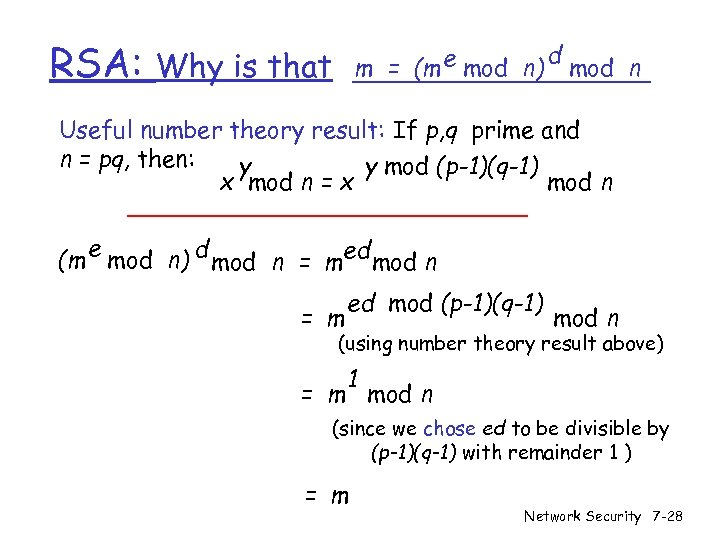RSA: m = (m e mod n) Why is that d mod n Useful number theory result: If p, q prime and n = pq, then: y y mod (p-1)(q-1) x mod n = x mod n e (m mod n) d mod n = med mod n = m ed mod (p-1)(q-1) mod n (using number theory result above) 1 = m mod n (since we chose ed to be divisible by (p-1)(q-1) with remainder 1 ) = m Network Security 7 -28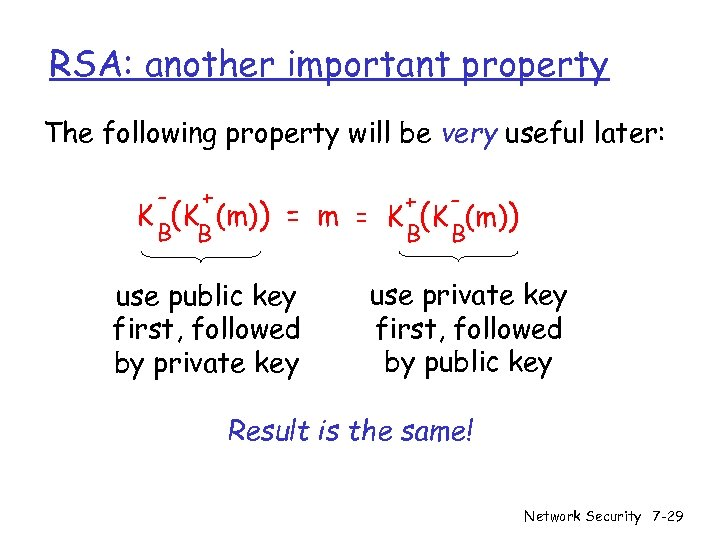RSA: another important property The following property will be very useful later: - + B B K (K (m)) + = m = K (K (m)) B B use public key first, followed by private key use private key first, followed by public key Result is the same! Network Security 7 -29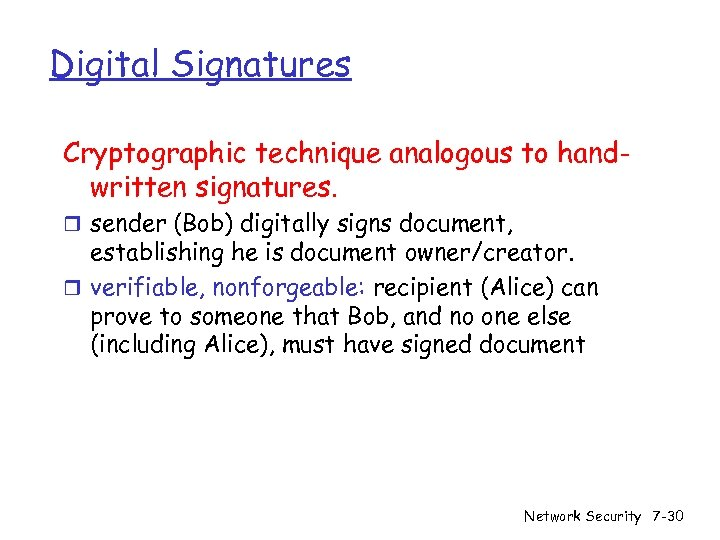Digital Signatures Cryptographic technique analogous to handwritten signatures. r sender (Bob) digitally signs document, establishing he is document owner/creator. r verifiable, nonforgeable: recipient (Alice) can prove to someone that Bob, and no one else (including Alice), must have signed document Network Security 7 -30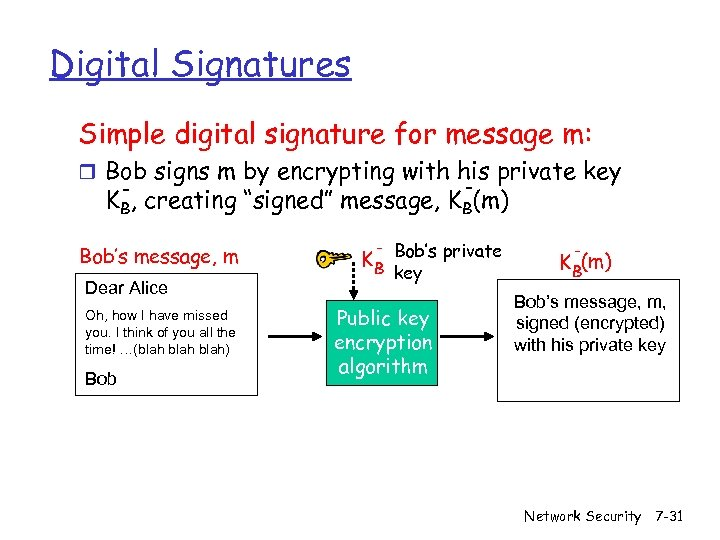Digital Signatures Simple digital signature for message m: r Bob signs m by encrypting with his private key - KB, creating “signed” message, KB(m) Bob’s message, m Dear Alice Oh, how I have missed you. I think of you all the time! …(blah) Bob K B Bob’s private key Public key encryption algorithm K B(m) Bob’s message, m, signed (encrypted) with his private key Network Security 7 -31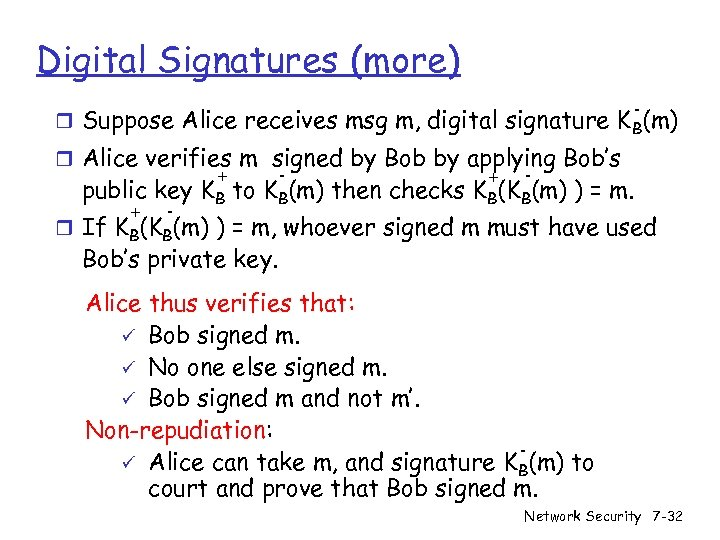Digital Signatures (more) - r Suppose Alice receives msg m, digital signature K B(m) r Alice verifies m signed by Bob by applying Bob’s + - public key KB to KB(m) then checks KB(KB(m) ) = m. + - r If KB(KB(m) ) = m, whoever signed m must have used Bob’s private key. Alice thus verifies that: ü Bob signed m. ü No one else signed m. ü Bob signed m and not m’. Non-repudiation: ü Alice can take m, and signature KB(m) to court and prove that Bob signed m. Network Security 7 -32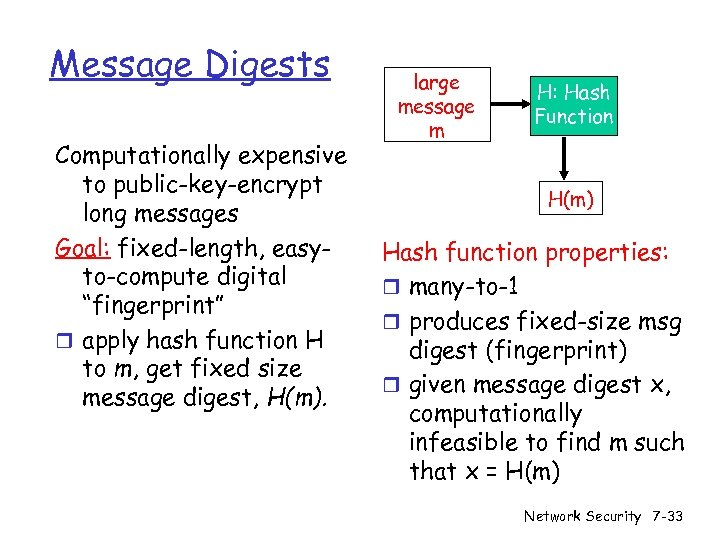Message Digests Computationally expensive to public-key-encrypt long messages Goal: fixed-length, easyto-compute digital “fingerprint” r apply hash function H to m, get fixed size message digest, H(m). large message m H: Hash Function H(m) Hash function properties: r many-to-1 r produces fixed-size msg digest (fingerprint) r given message digest x, computationally infeasible to find m such that x = H(m) Network Security 7 -33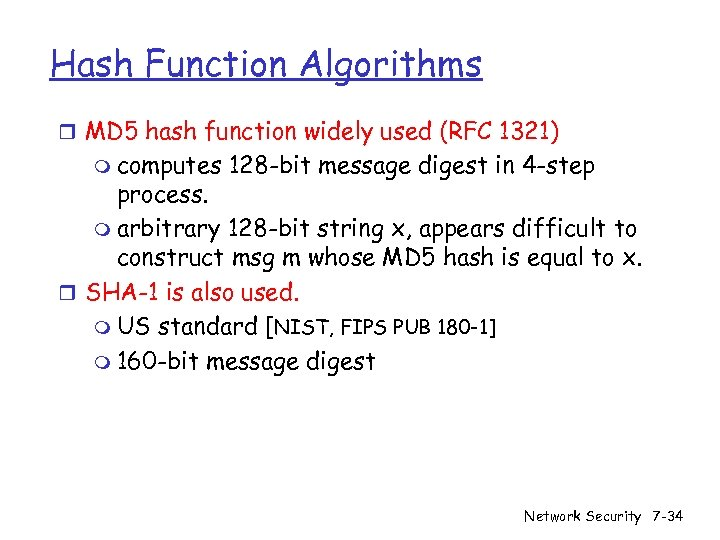Hash Function Algorithms r MD 5 hash function widely used (RFC 1321) m computes 128 -bit message digest in 4 -step process. m arbitrary 128 -bit string x, appears difficult to construct msg m whose MD 5 hash is equal to x. r SHA-1 is also used. m US standard [NIST, FIPS PUB 180 -1] m 160 -bit message digest Network Security 7 -34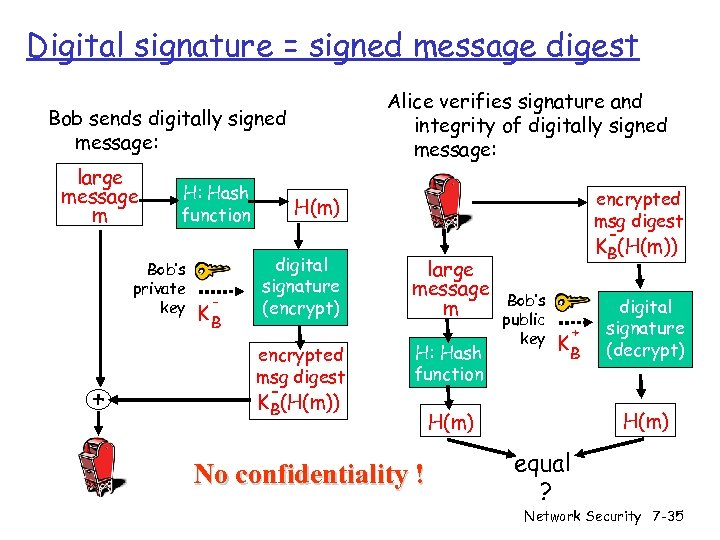Digital signature = signed message digest Alice verifies signature and integrity of digitally signed message: Bob sends digitally signed message: large message m H: Hash function Bob’s private key + - KB encrypted msg digest H(m) digital signature (encrypt) encrypted msg digest KB(H(m)) large message m H: Hash function No confidentiality ! KB(H(m)) Bob’s public key + KB digital signature (decrypt) H(m) equal ? Network Security 7 -35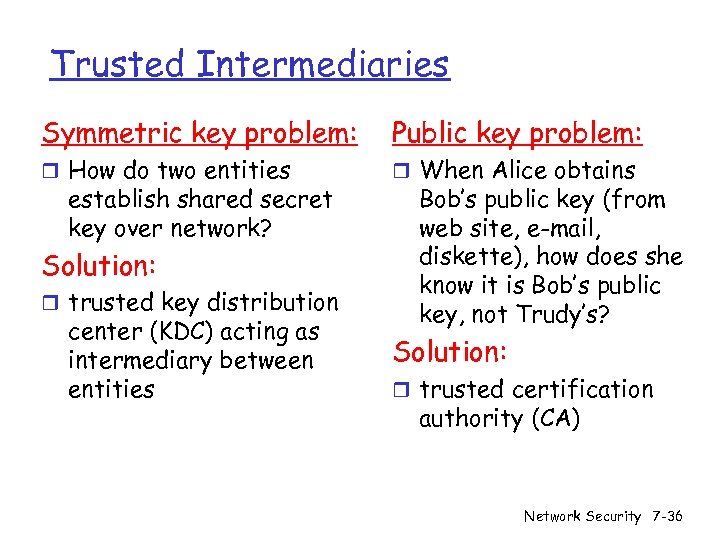Trusted Intermediaries Symmetric key problem: Public key problem: r How do two entities r When Alice obtains establish shared secret key over network? Solution: r trusted key distribution center (KDC) acting as intermediary between entities Bob’s public key (from web site, e-mail, diskette), how does she know it is Bob’s public key, not Trudy’s? Solution: r trusted certification authority (CA) Network Security 7 -36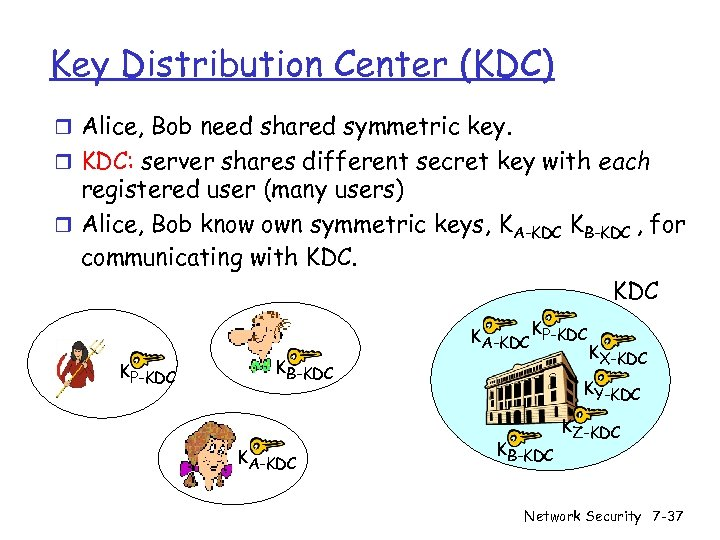Key Distribution Center (KDC) r Alice, Bob need shared symmetric key. r KDC: server shares different secret key with each registered user (many users) r Alice, Bob know own symmetric keys, KA-KDC KB-KDC , for communicating with KDC KA-KDC KP-KDC KB-KDC KA-KDC KX-KDC KY-KDC KB-KDC KZ-KDC Network Security 7 -37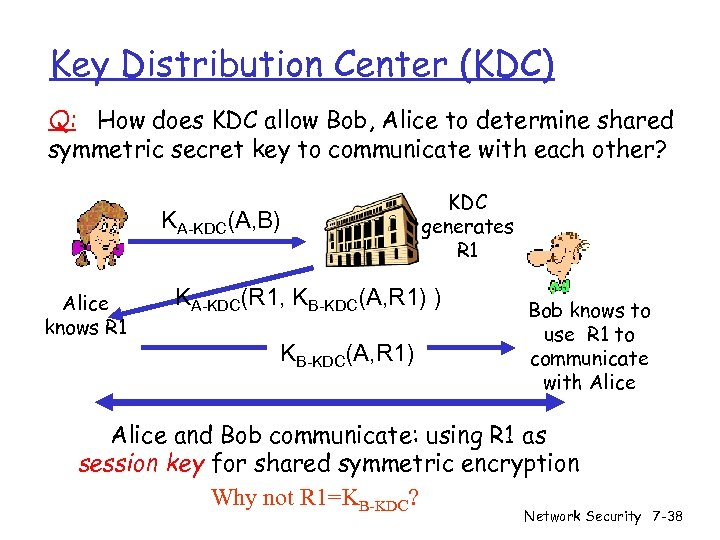Key Distribution Center (KDC) Q: How does KDC allow Bob, Alice to determine shared symmetric secret key to communicate with each other? KA-KDC(A, B) Alice knows R 1 KDC generates R 1 KA-KDC(R 1, KB-KDC(A, R 1) ) KB-KDC(A, R 1) Bob knows to use R 1 to communicate with Alice and Bob communicate: using R 1 as session key for shared symmetric encryption Why not R 1=KB-KDC? Network Security 7 -38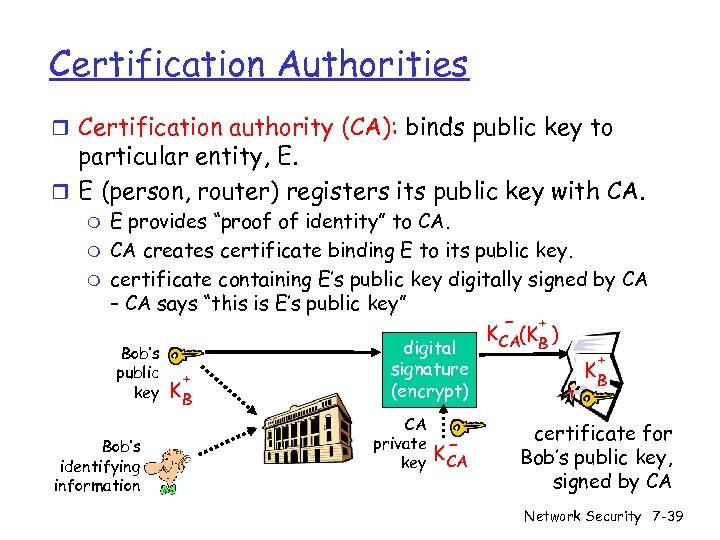Certification Authorities r Certification authority (CA): binds public key to particular entity, E. r E (person, router) registers its public key with CA. m m m E provides “proof of identity” to CA. CA creates certificate binding E to its public key. certificate containing E’s public key digitally signed by CA – CA says “this is E’s public key” - + K CA(KB ) Bob’s public key Bob’s identifying information + KB digital signature (encrypt) CA private key K- CA + KB certificate for Bob’s public key, signed by CA Network Security 7 -39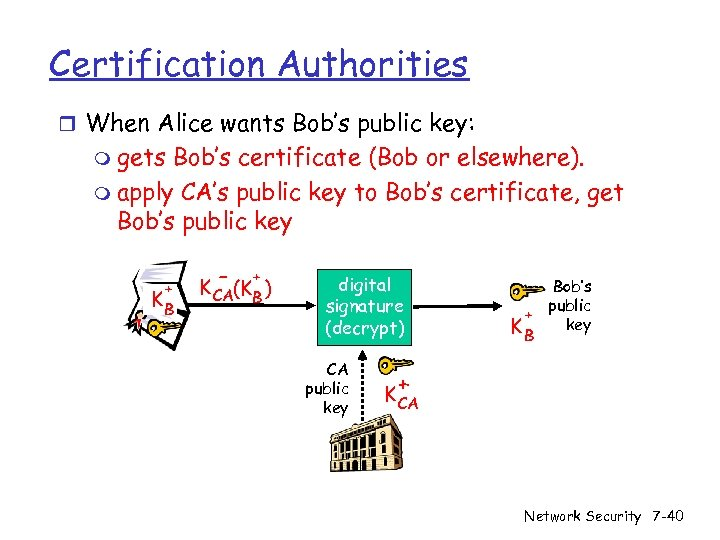Certification Authorities r When Alice wants Bob’s public key: m gets Bob’s certificate (Bob or elsewhere). m apply CA’s public key to Bob’s certificate, get Bob’s public key + KB - + K CA(KB ) digital signature (decrypt) CA public key Bob’s public + key KB + K CA Network Security 7 -40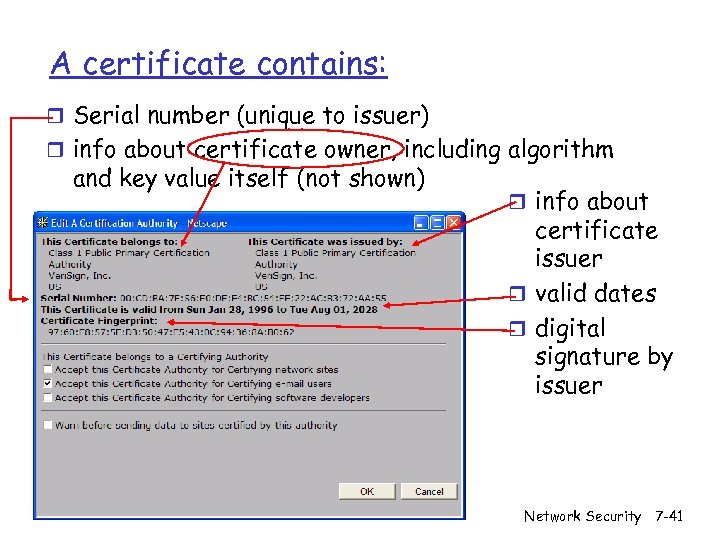A certificate contains: r Serial number (unique to issuer) r info about certificate owner, including algorithm and key value itself (not shown) r info about certificate issuer r valid dates r digital signature by issuer Network Security 7 -41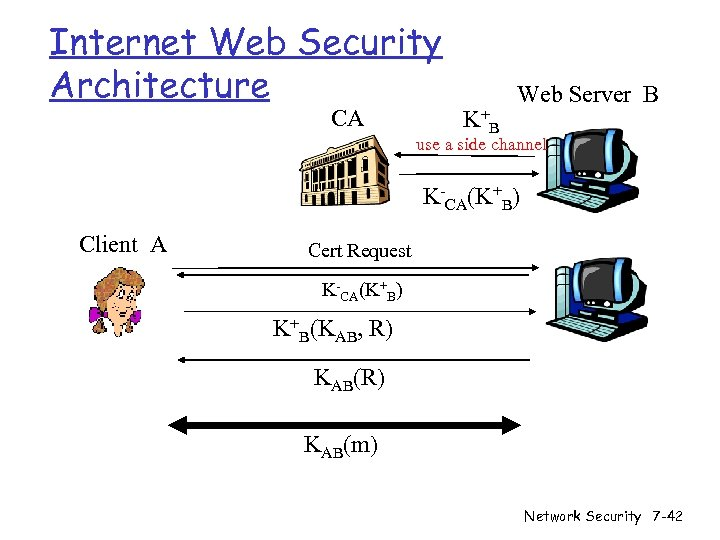Internet Web Security Architecture CA K+ B Web Server B use a side channel K-CA(K+B) Client A Cert Request K-CA(K+B) K+B(KAB, R) KAB(m) Network Security 7 -42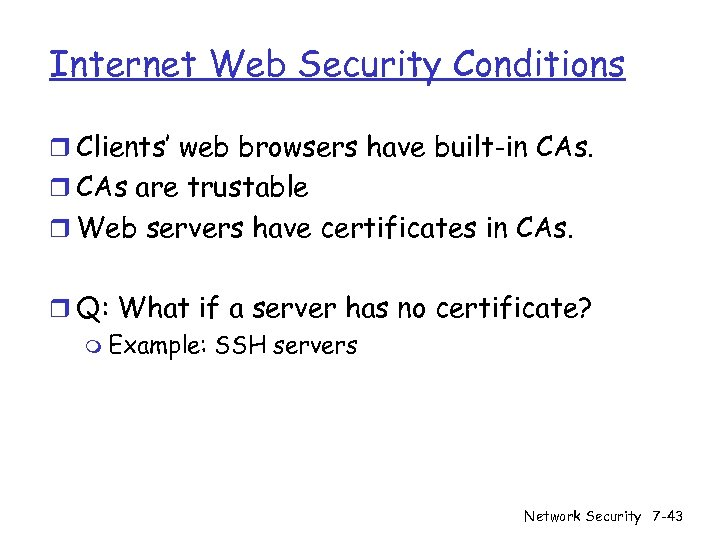Internet Web Security Conditions r Clients’ web browsers have built-in CAs. r CAs are trustable r Web servers have certificates in CAs. r Q: What if a server has no certificate? m Example: SSH servers Network Security 7 -43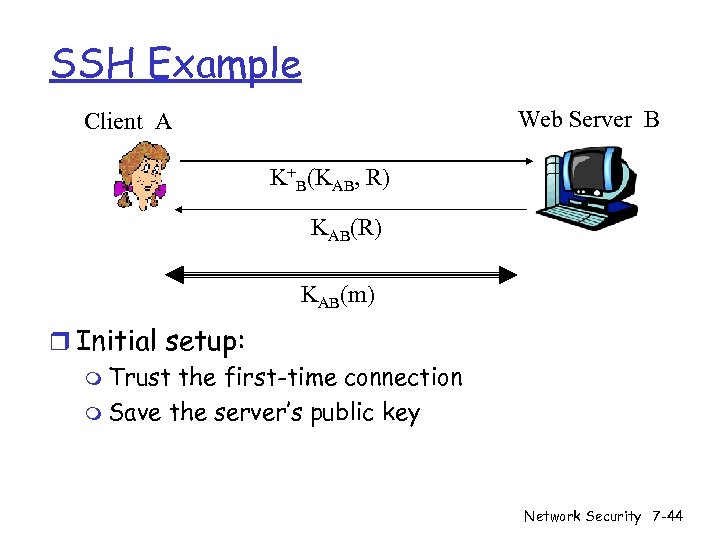SSH Example Web Server B Client A K+B(KAB, R) KAB(m) r Initial setup: m Trust the first-time connection m Save the server’s public key Network Security 7 -44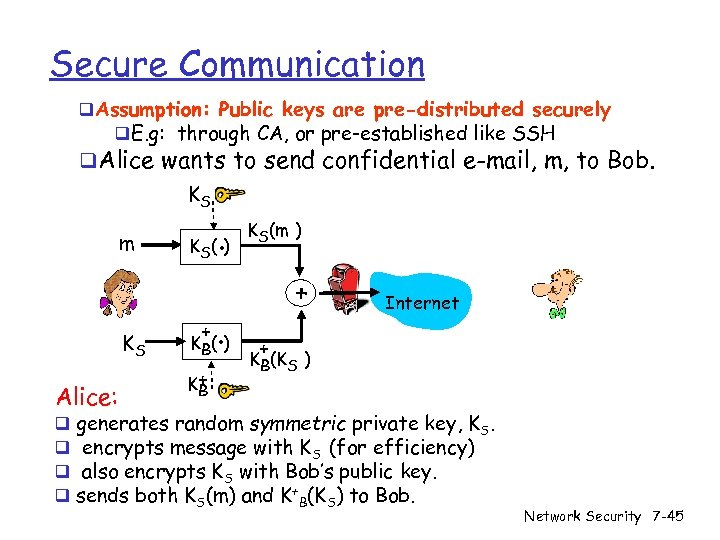Secure Communication q. Assumption: Public keys are pre-distributed securely q. E. g: through CA, or pre-established like SSH q. Alice wants to send confidential e-mail, m, to Bob. KS m KS Alice: q q . K S( ) + . K B( ) K+ B KS(m ) + Internet + KB(KS ) generates random symmetric private key, KS. encrypts message with KS (for efficiency) also encrypts KS with Bob’s public key. sends both KS(m) and K+B(KS) to Bob. Network Security 7 -45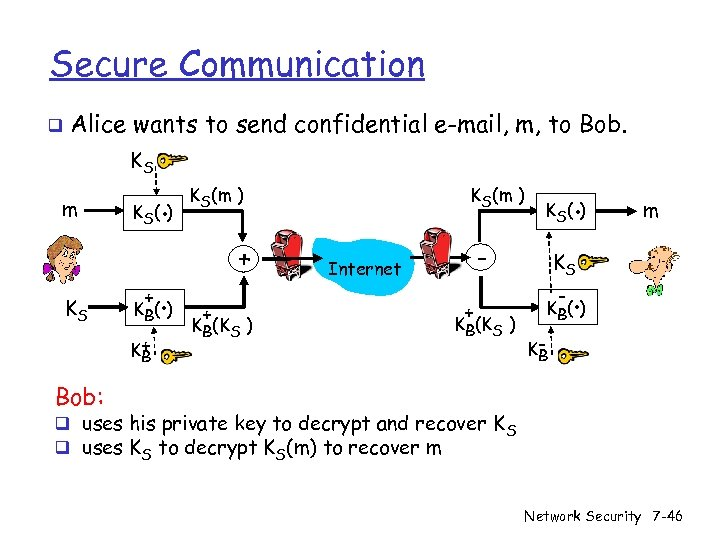Secure Communication q Alice wants to send confidential e-mail, m, to Bob. KS m KS K (. ) S + . K B( ) K+ B KS(m ) + + KB(KS ) . K S( ) - Internet + KB(KS ) m KS - . K B( ) KB Bob: q uses his private key to decrypt and recover K S q uses KS to decrypt KS(m) to recover m Network Security 7 -46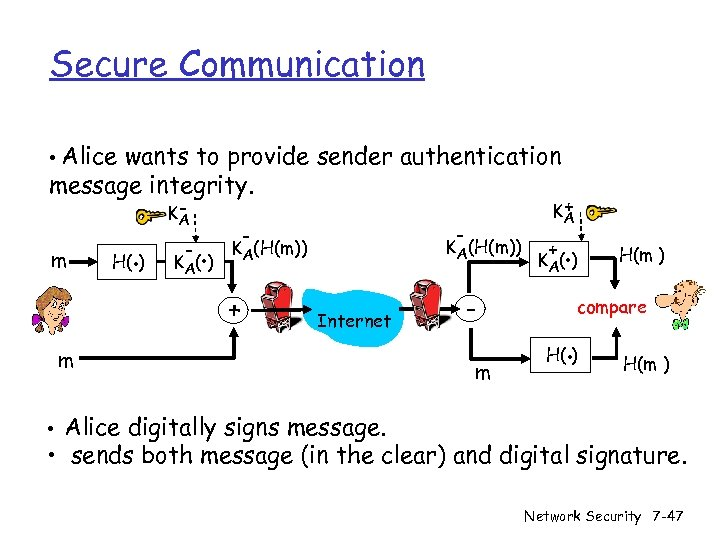Secure Communication • Alice wants to provide sender authentication message integrity. m H(. ) KA - . K A( ) - - KA(H(m)) + + KA Internet m m + . K A( ) H(m ) compare . H( ) H(m ) • Alice digitally signs message. • sends both message (in the clear) and digital signature. Network Security 7 -47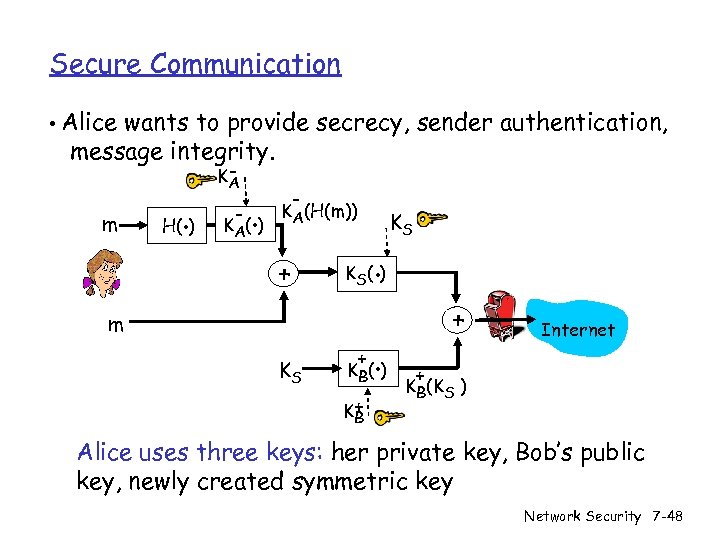Secure Communication • Alice wants to provide secrecy, sender authentication, message integrity. m . H( ) KA - . K A( ) - KA(H(m)) + . K S( ) m KS KS + . K B( ) K+ B + Internet + KB(KS ) Alice uses three keys: her private key, Bob’s public key, newly created symmetric key Network Security 7 -48